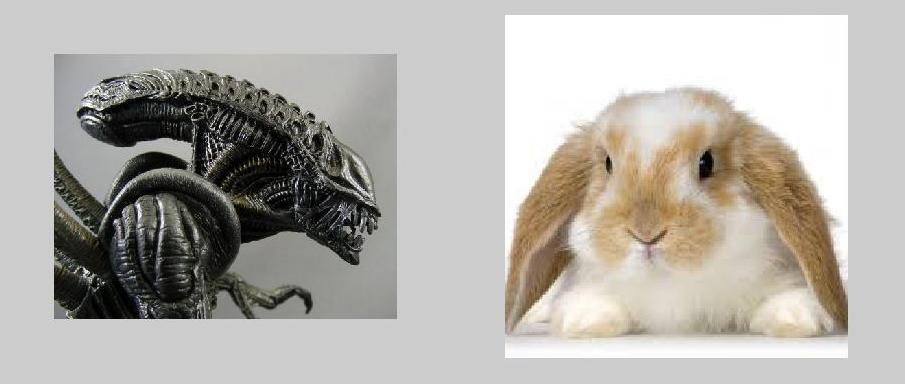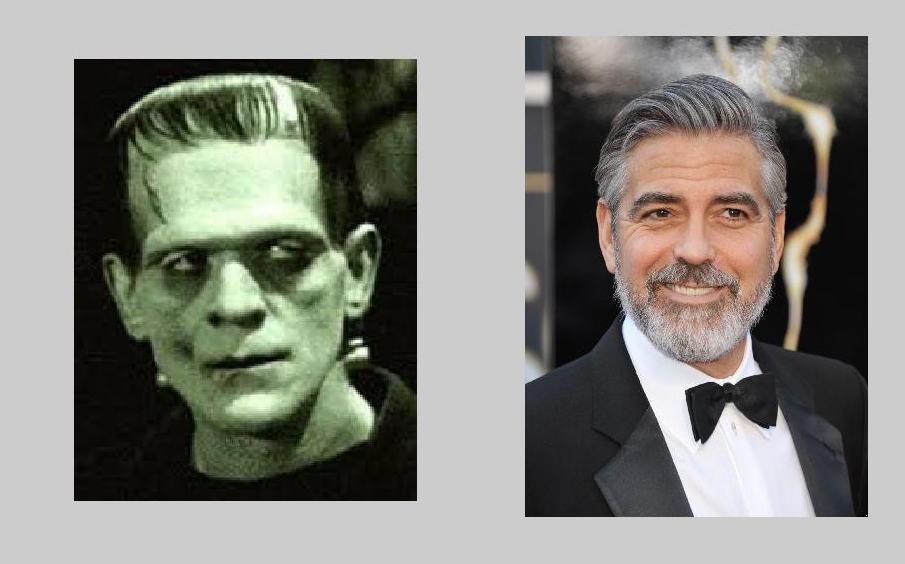# Reverse Singular Value Decomposition

Employing a factorization based on the least significant singular values provides a matrix approximation with many surprisingly useful properties. This Reverse Singular Value Decomposition, RSVD, is also referred to as Subordinate Component Analysis, SCA, to distinguish it from Principal Component Analysis.

### Contents

#### RSVD

The Singular Value Decomposition of a matrix $A$ is computed by
    [U,S,V] = svd(A);
This generates two orthogonal matrices $U$ and $V$ and a diagonal matrix $S$ with diagonal elements $\sigma_k$ that provide the expansion $$A = \sigma_1 E_1 + \sigma_2 E_2 + ... + \sigma_n E_n$$ where $E_k$ is the rank one outer product $$E_k = U(:,k) V(:,k)'$$ Traditionally, the singular values are arranged in descending order. In contrast, the Reverse Singular Value Decomposition Approximation of rank $r$ is obtained by arranging the singular values in ascending order, $$0 \le \sigma_1 \le \sigma_2 \le ...$$ and then using the first $r$ terms from the expansion. Here is a function that computes the RSVD for square or rectangular matrices with at least as many rows as columns.
   type rsvd

function X = rsvd(A,r)
% RSVD  Approximation by the Reverse Singular Value Decomposition
%   rsvd(A,r) approximates A by a matrix of rank r obtained from
%   the r least significant singular values in ascending order.

[m,n] = size(A);
[U,S,V] = svd(A,'econ');
k = n:-1:n-r+1;
X = U(:,k)*S(k,k)*V(:,k)';


#### Roundoff Error

In certain situations, the RSVD can reduce or even eliminate roundoff error. For example, according to its help entry the elmat function hilb attempts to compute
    hilb(N) is the N by N matrix with elements 1/(i+j-1)
But the function can only succeed to within roundoff error. Its results are binary floating point numbers approximating the reciprocals of integers described in the help entry. Here is the printed output with n = 5 and the default format short.
   format short
H = hilb(5)

H =

1.0000    0.5000    0.3333    0.2500    0.2000
0.5000    0.3333    0.2500    0.2000    0.1667
0.3333    0.2500    0.2000    0.1667    0.1429
0.2500    0.2000    0.1667    0.1429    0.1250
0.2000    0.1667    0.1429    0.1250    0.1111


We are seeing the effects of the output conversion as well as the underlying binary approximation. Perhaps surprisingly, the reverse singular value decomposition can eliminate both sources of error and produce the exact rational entries of the theoretical Hilbert matrix.
   format rat
H = rsvd(H,5)

H =

1              1/2            1/3            1/4            1/5
1/2            1/3            1/4            1/5            1/6
1/3            1/4            1/5            1/6            1/7
1/4            1/5            1/6            1/7            1/8
1/5            1/6            1/7            1/8            1/9



#### Text Processing

The RSVD is capable of uncovering spelling and typographical errors in text. My web site for Numerical Computing with MATLAB has a file with the text of Lincoln's Gettysburg Address. gettysburg.txt I've used this file for years whenever I want to experiment with text processing. You can download the file and then use the MATLAB data import wizard with column delimeters set to spaces, commas and periods to produce a cell array, gettysburg, of individual words. There are 278 words. The longest word has 11 characters. So
    A = cell2mat(gettysburg)
converts the cell array of strings to a 278-by-11 matrix of doubles. It turns out that an RSVD approximation of rank nine finds three spelling errors in the original text.
    B = rsvd(A,9);
k = find(sum(A,2)~=sum(B,2))
disp([char(A(k,:))  char(B(k,:))])
    weather      whether
consicrated  consecrated
govenment    government
In all the years that I have been using this data, I have never noticed these errors.

#### Image Processing

We have also found that the RSVD is capable of softening the appearance of aggression in photographs. A matrix is obtained from the JPEG image by stacking the RGB components vertically. Roughly 90% of the small singular values then produce a pleasant result.
    RGB = imread('creature.jpg');
A = [RGB(:,:,1); RGB(:,:,2); RGB(:,:,3)];
[m,n] = size(A);
B = rsvd(A,ceil(0.90*n));
m = m/3;
C = cat(3,B(1:m,:),B(m+1:2*m,:),B(2*m+1:3*m,:))
image(C)Published with MATLAB® R2014a

|